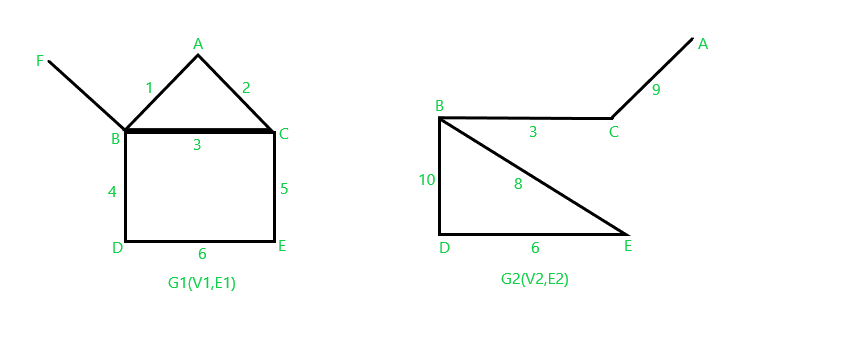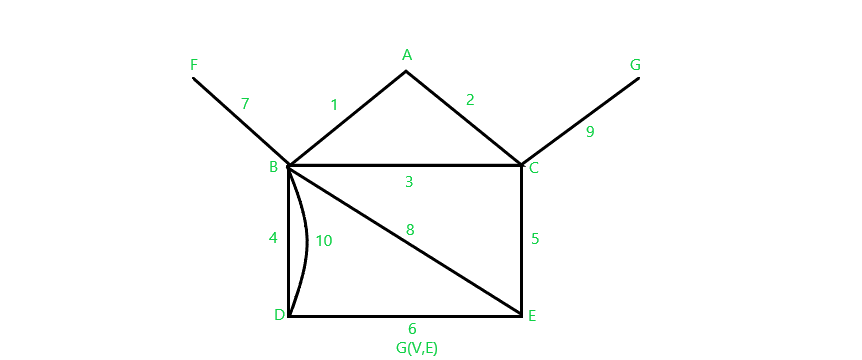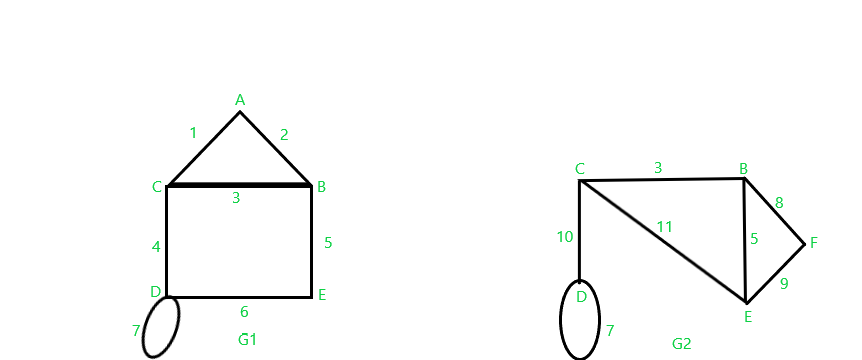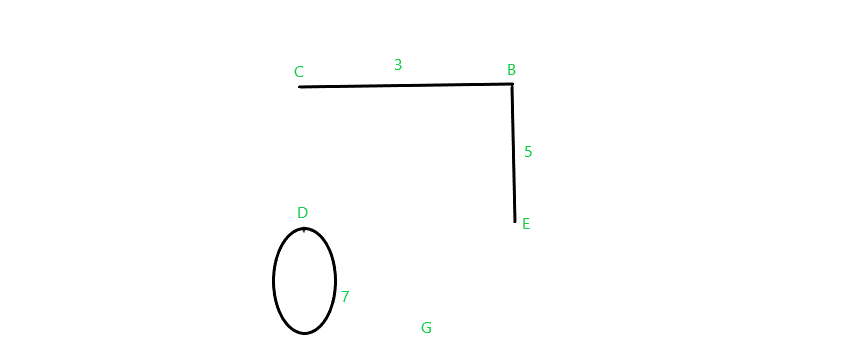Open in App
Not now

# Union and Intersection Operation On Graph

• Last Updated : 30 Jan, 2023

In graph theory, the data/objects belong to the same group but each piece of data differs from one other. In this article, we will see union and intersection operations on the graph.

## Union Operation

Let G1(V1, E1) and G2(V2, E2) be two graphs as shown below in the diagram. The union of G1 and G2 is a graph G=G1∪G2, where vertex set V=V1 ∪ V2 and edge set E= E1 ∪ E2For the above two graphs G1 and G2, we have vertices and edges as V1= { A, B, C, D, E, F } and E1= { 1,2,3,4,5,6,7 } and V2= {B, C, D, E, G} and E2= {3,6,8,9,10} respectively.

So, in order to find the union of graphs G1 and G2, which can be denoted as G= G1∪G2. The vertex set of graph G will be V=V1∪V2= {A, B, C , D, E, F, G} and the edge set of graph G will be E=E1∪E2 ={1,2,3,4,5,6,7,8,9,10}.

The resultant union graph G with all the vertices of set V and edges of set E will be as shown:Be careful to retain the original order of vertices and edges as in the original graph.

## Intersection Operation

Let G1(V1,E1) and G2(V2,E2) be two graphs. Then the intersection of G1 and G2 is a graph G=G1∩G2, whose vertex set V=V1∩V and edge set E=E1∩E2.For the above two graphs G1 and G2, we have vertices and edges as V1= { A, B, C, D, E } and E1= { 1,2,4,5,6,7 } and V2= {B, C, D, E, F} and E2= {3,5,7,8,9,10,11} respectively.

So, in order to find the intersection of graphs G1 and G2, which can be denoted as G= G1∩G2. The vertex set of graph G will be V=V1∩V2= {B, C, D, E} and the edge set of graph G will be E=E1∩E2 ={3,5,7}.

The resultant intersection graph G with all the vertices of set V and edges of set E will be as shown:Be careful to retain the original order of vertices and edges as in the original graph in the resultant graph.

My Personal Notes arrow_drop_up
Related Articles